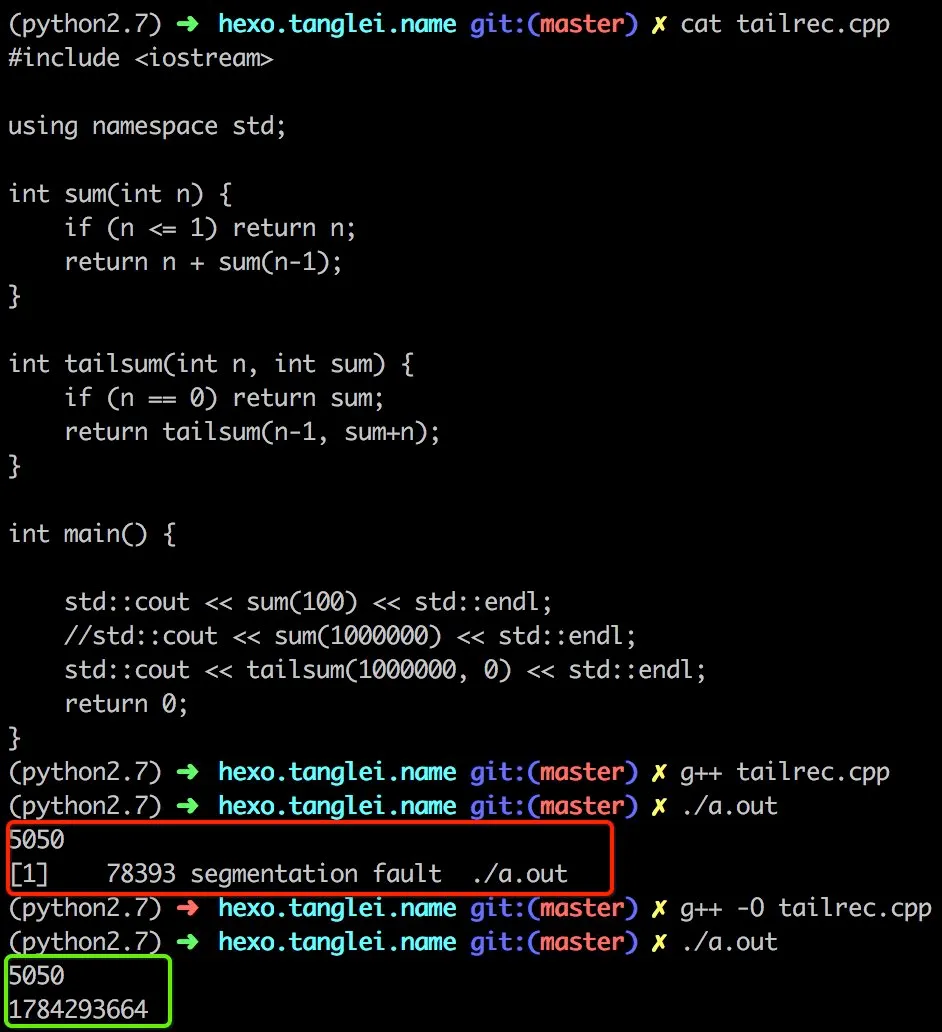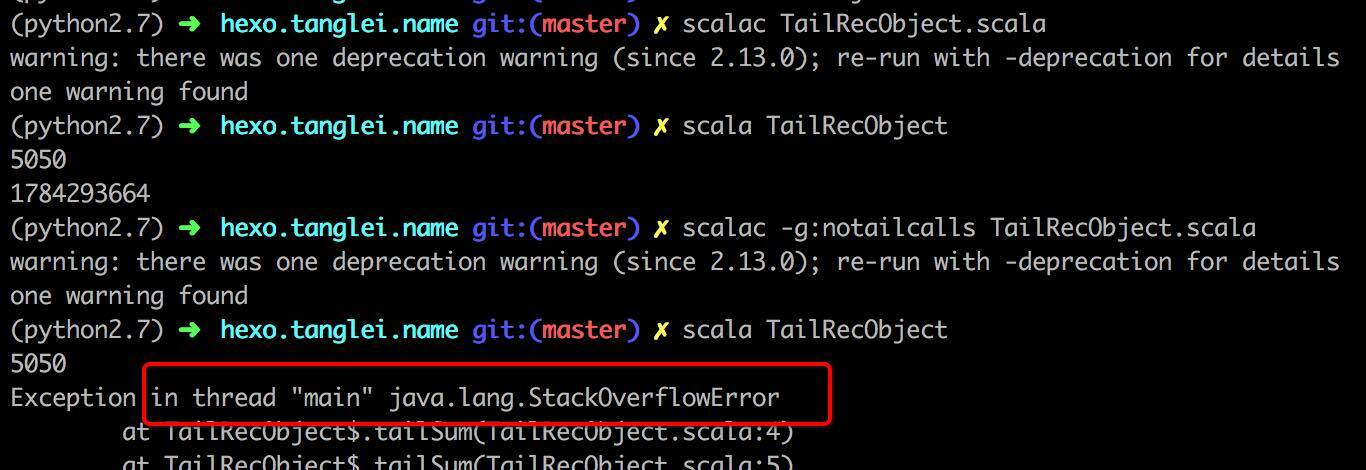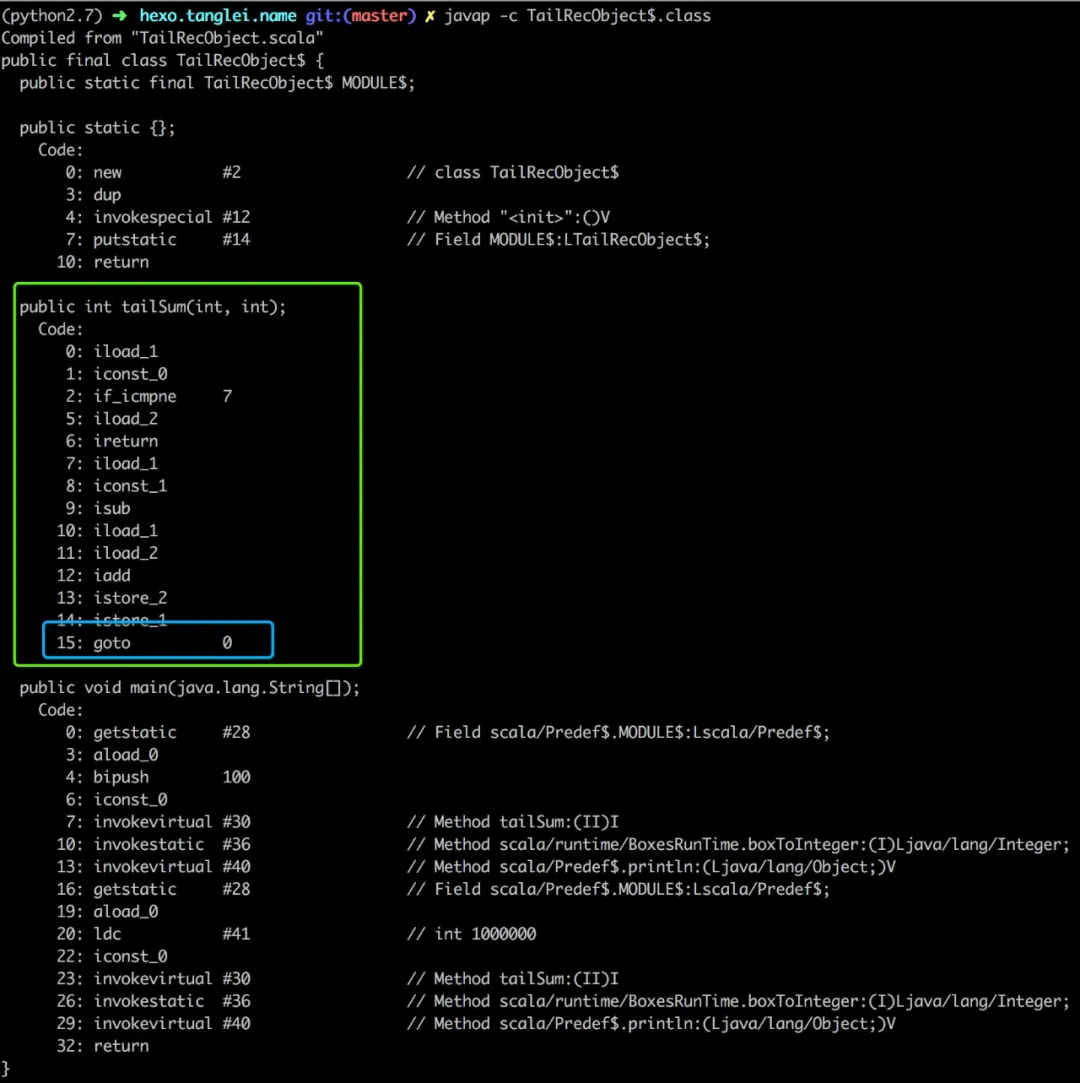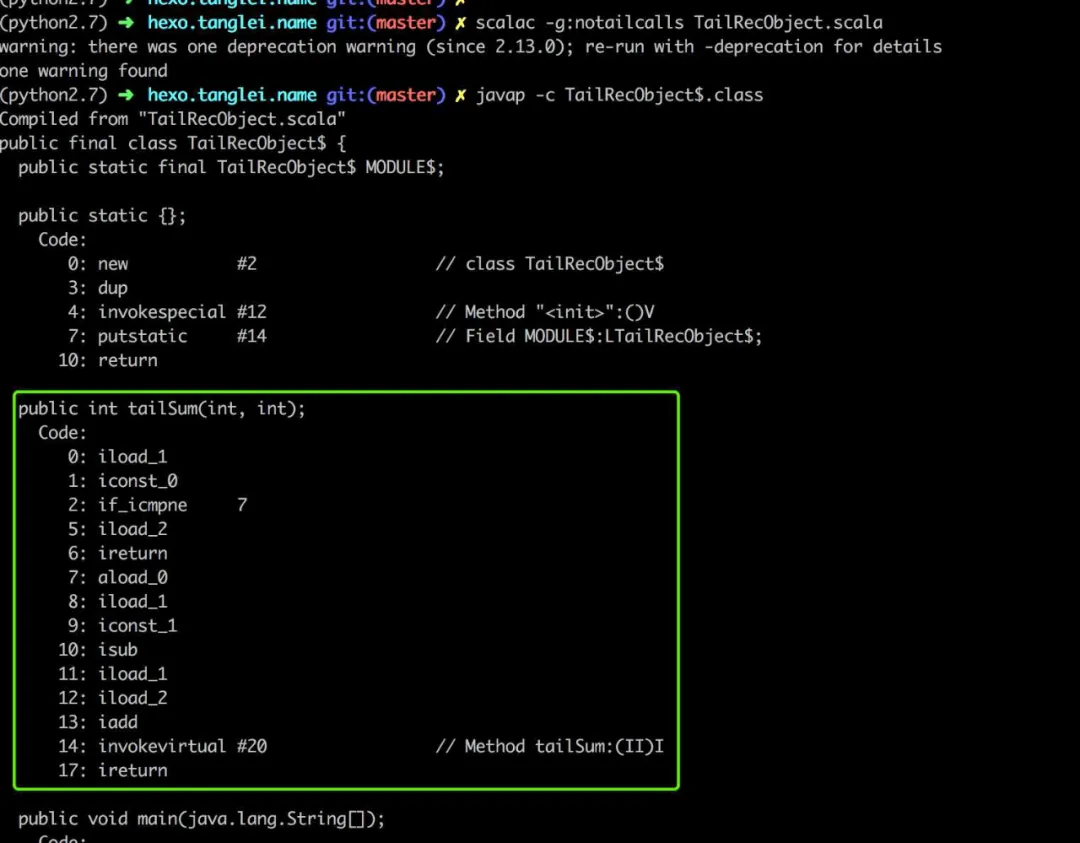# 大家都知道递归，尾递归呢？什么又是尾递归优化？﻿

## 递归是啥

﻿

﻿

int Fibonacci(n) {	if (n < 2) return n;	return Fibonacci(n - 1) + Fibonacci(n - 2);}

﻿

﻿

﻿

﻿

int sum(int n) {    if (n <= 1) return n;    return n + sum(n-1);}

﻿

std::cout << sum(100) << std::endl;std::cout << sum(1000000) << std::endl;

﻿

﻿

(python2.7) ➜  hexo.tanglei.name git:(master) ✗ ./a.out5050    78159 segmentation fault  ./a.out

﻿

## 尾递归又是啥？

﻿

﻿

int tailsum(int n, int sum) {    if (n == 0) return sum;    return tailsum(n-1, sum+n);}

﻿

﻿

## 尾递归优化

﻿

﻿﻿

﻿

object TailRecObject {   def tailSum(n: Int, sum: Int): Int = {        if (n == 0) return sum;        return tailSum(n-1, n+sum);    }   def main(args: Array[String]) {      println(tailSum(100, 0))      println(tailSum(1000000, 0))   }}

﻿

﻿﻿

﻿﻿﻿

﻿

﻿

﻿## 评论